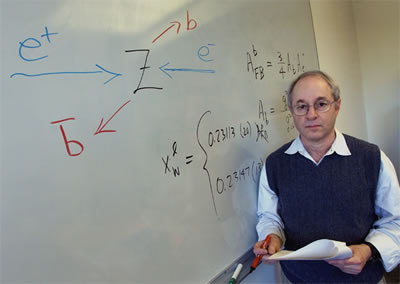Michael Chanowitz is a theoretical physicist at Lawrence Berkeley National Laboratory. He is the author with Mary K. Gaillard of the heavily cited 1985 paper entitled: "The TeV Physics of Strongly Interacting W's and Z's." While ATLAS and CMS are narrowing the allowed mass regions where a Higgs boson may be found, Chanowitz addresses what would be the impact of not finding the Higgs. (Image: ATLAS Experiment)

Physicists are confident they will soon be able to answer a fundamental question at the LHC: how do particles acquire mass? The simplest answer, the one given in the Standard Model of the fundamental particles, is that a single particle, the Higgs boson, endows the other particles and itself with mass. The Higgs boson does this by means of the "Higgs mechanism", which involves breaking a symmetry that would leave all Standard Model particles massless if it were not broken.

### However the Higgs boson is not the only way the Higgs mechanism might work.

It might instead work by a yet unknown collection of very heavy particles interacting by means of a yet unknown strong force. This possibility is referred to as "dynamical symmetry breaking". In this case, there would be no Higgs boson.

Without understanding the origin of mass, the theory of fundamental particles is not only incomplete but is truly nonsensical, because it predicts that certain processes occur with probability greater than 100%. The Higgs mechanism eliminates this nonsense from the theory at the same time that it explains how mass is acquired.

But the nonsensical prediction of the incomplete theory is actually very useful, because it allows us to determine the energy and collision rate needed by a particle accelerator to discover the true nature of the Higgs mechanism, whether by Higgs boson or dynamical symmetry breaking.

A central role in the LHC's search for the mechanism of mass generation is played by the W boson. The W boson is the massive force carrier of the weak nuclear force that causes radioactivity. Without the Higgs mechanism the incomplete theory predicts that the collision rate for two W bosons to collide increases without limit as their energy increases. The increase in collision rate is known to be true at low energy but would nonsensically exceed 100% probability if it continued to very high energy.

In the Standard Model the Higgs boson generates a force between the two W bosons that stops the growth of the WW collision rate before it gets close to the 100% maximum. But if the Higgs mechanism is realized by dynamical symmetry breaking, the collision probability will grow until it is near the 100% maximum, where its growth is halted by the action of the new particles that generate the symmetry breaking force. In both cases the force that arrests the growth of the collision rate is also responsible for generating the W boson mass.

The Higgs boson is expected to have a mass below 1 TeV (one trillion electron-volts) and will stop the growth of the WW collision rate at an energy equal to its mass, that is, at the same energy at which it can be produced. Particles responsible for dynamical symmetry breaking are expected to be heavier than 1 TeV and freeze the collision rate at an energy above 1 TeV but below about 2 TeV where the 100% maximum would be exceeded. A collision rate near the maximum is called "strong WW scattering", while a lower collision rate denotes "weak scattering" ("scattering" refers to the collision of two W bosons). If strong WW scattering occurs, then additional W boson pairs are produced at very high energy.

If we are able to detect the additional W boson pairs that are the signal of strong WW scattering, we can determine by the presence or absence of the signal, whether the Higgs mechanism is implemented by a Higgs boson or dynamically. Absence of the signal would imply a Higgs boson (or bosons) below 1 TeV, while presence of the signal would imply dynamical symmetry breaking by particles above 1 TeV.

This dichotomy gives rise to the "No-Lose Theorem": an accelerator with enough energy and intensity to be sensitive to strong WW scattering can reveal the nature of the mass generating mechanism whether strong WW scattering is observed or not. At its design energy and intensity the LHC is a "no-lose" accelerator. If strong WW scattering is not observed at the LHC, we are directed to focus the search on a "light" Higgs boson below 1 TeV. A light Higgs boson might have eluded early discovery because it decays in unexpected ways.

If a Higgs boson is discovered, we should confirm the absence of strong WW scattering to verify the Higgs mechanism. If a Higgs boson is not discovered and strong WW scattering is observed, it would direct us to focus the search above 1 TeV, which will likely require increasing the LHC energy and intensity.

There is still one other possibility, which we might call "none of the above". The Higgs mechanism has not yet been confirmed by direct observation, and although it would shock most physicists, it is possible that mass is generated in some other way. In this case we also expect that strong WW scattering will occur, and it will be very challenging to differentiate from dynamical symmetry breaking. Even in this case, the LHC will guide by restricting the possibilities, enabling us to plan the program that will lead to the answers.

The study of strong WW scattering, its presence or absence, is very challenging, pushing the accelerator to the limits of its capability. It is however well worth the effort, because it is fundamental to understanding how mass is generated. Because of the ability to observe strong WW scattering, we are confident the LHC will show us the way to uncover the deep mystery of the origin of mass.